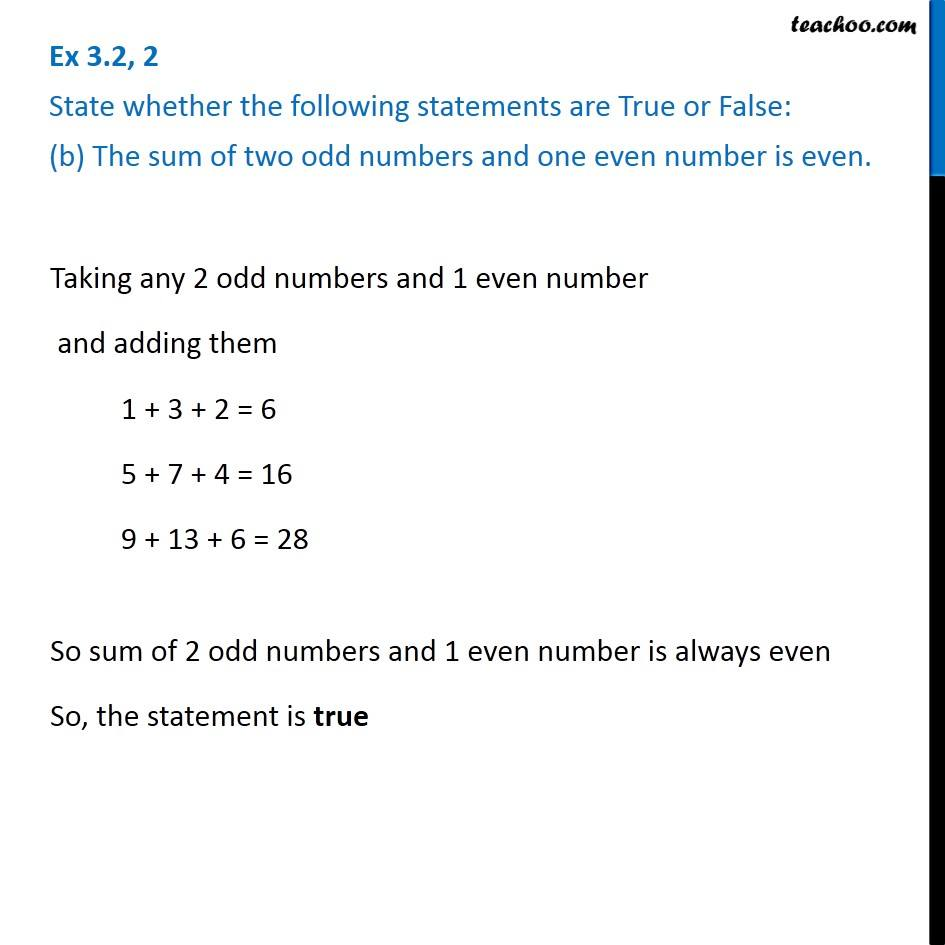1. Chapter 3 Class 6 Playing with Numbers
2. Serial order wise
3. Ex 3.2

Transcript

Ex 3.2, 2 State whether the following statements are True or False: (b) The sum of two odd numbers and one even number is even. Taking any 2 odd numbers and 1 even number and adding them 1 + 3 + 2 = 6 5 + 7 + 4 = 16 9 + 13 + 6 = 28 So sum of 2 odd numbers and 1 even number is always even So, the statement is true